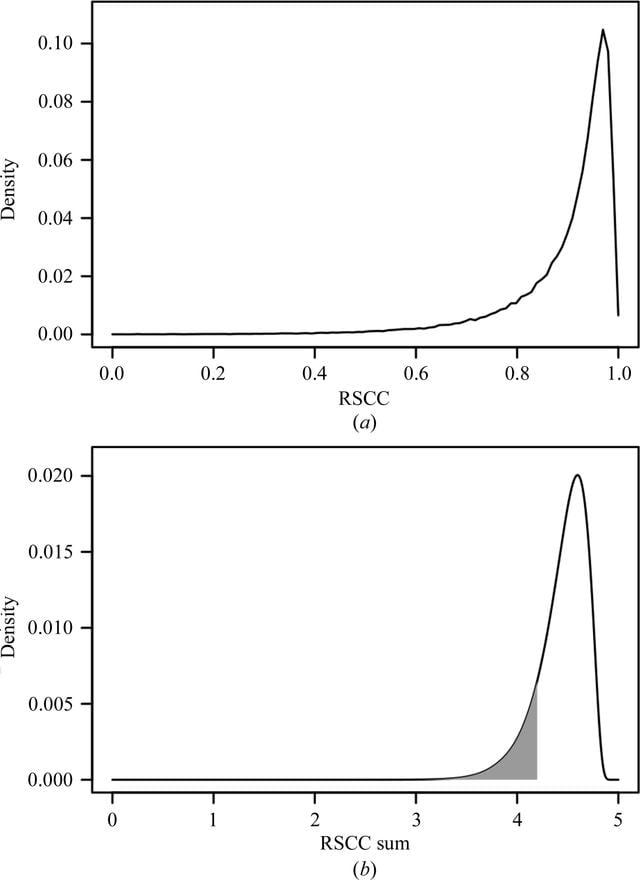disable zoom     view article Figure 1 Probability density functions of RSCC values and sums. (a) Graph of f1, the empirical p.d.f. of RSCC values derived from 112 565 residues belonging to peptide-ligand chains, with the bin size set to 0.01. (b) Graph of f5, which is the p.d.f. of RSCC sums S5, calculated by iterative convolutions of f1. The grey area represents P(Sn ≤ 4.2), the probability of observing a sum of five RSCC values less than or equal to 4.2, which is given by integrating f5 from −∞ to 4.2, which is equivalent to evaluating the respective cumulative distribution function F(4.2) = 0.1426. The distribution of the random variable S5 peaks at 4.6 and F(4.6) = P(Sn ≤ 4.6) = 0.71.STRUCTURALBIOLOGY
ISSN: 2059-7983
Volume 73| Part 3| March 2017| Pages 211-222